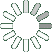Puzzle Command
Daily Puzzle
Number Logic #18
March 1, 2015Find a 6 digit number where:

The 1st digit is equal to 1.
The 2nd digit is equal to 9.
The 3rd digit is not equal to 8 and is even.
The 4th digit is the sum of the 1st and 3rd digit and equal to 1.
The 5th digit is the sum of the 4th and 6th digit.
The 6th digit is a prime number and a multiple of 2.

Puzzle Notes
6 digit numbers range from 100,000 to 999,999.
Enter in your solution below and click submit.